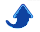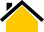# Dr. Phillip M. Feldman

In reading the following, bear in mind that I used Matlab for almost 20 years before making the switch to Python in 2009, so I am intimately familiar with both. I would estimate that I reached the "productivity crossover point" after using Python for roughly six weeks. Although Python and Matlab are comparable in several areas, Python offers several important advantages.

## Advantage #1: Python code tends to be more compact and readable than Matlab code.

There are several reasons for this:
1. Unlike Matlab, which uses `end` statements as closures (i.e., to indicate the end of a block), Python determines the scope of a block based on its indentation. This forces Python programmers to indent code blocks, which is good practice anyway. (And, unlike programmers working in most other languages, no Python programmer ever wastes time hunting for a missing `end` statement).

2. Like almost every programming language other than Matlab, Python uses square brackets for indexing and parentheses for function and method calls. Matlab uses parentheses for both. Python's use of square brackets for indexing is important for readability, and also makes life easier for programmers who must work with multiple languages. Matlab's use of parentheses for both indexing and function calls can make Matlab code hard to understand and is a frequent source of bugs.

3. Support for augmented assignments tends to make Python code less verbose than Matlab code. Compare the following two fragments of code, which collect counts for a 2-D histogram:

Matlab:

``` count(max(floor(x),1), max(floor(y),1))= ... count(max(floor(x),1), max(floor(y),1)) + 1 ```

Python:

``` count[max(floor(x),0), max(floor(y),0)]+= 1 ```

Note that the Matlab code is roughly twice as long. The chances of a typographical error are greater when entering this code, and it takes longer for someone to read and understand it. In general, Python code is almost always smaller (in terms of lines of code, assuming only one statement and no more than 80 characters per line) than Matlab code that performs the same task. For code that performs primarily numerical operations, one can expect a Python implementation to be 10 to 20 percent smaller than equivalent Matlab code. For programs that perform extensive string processing, the advantage of Python is even greater.

4. Python/NumPy's array methods `min`, `max`, `mean`, etc. operate by default on all dimensions of an array. Compare the following two fragments of code that calculate the mean of a 4-D array.

Matlab:

``` m= mean(mean(mean(mean(x)))); ```

Python:

``` m= mean(x) ```
5. Python does not arbitrarily restrict the use of literals in expressions. Here is some sample input/output from an IPython session:

``` In: n=3 In: [1,2,4,7,11,14,16,17][n] Out: 7 ```

Now try the same thing in Matlab:

``` >> n= 4 % Matlab indices start from 1 >> [1,2,4,7,11,14,16,17](n) Error: Unbalanced or unexpected parenthesis or bracket ```

The better readability of Python is much more than a matter of aesthetics. Better readability leads to fewer bugs, faster debugging when bugs are introduced, and faster comprehension when one must work with someone else's code (or with one's own code after a long hiatus).

## Advantage #2: Like almost every programming language other than Matlab, Python uses zero-based indexing

To illustrate, let `x` be a one-dimensional array. With Python/NumPy, the first element is `x`—not `x`. Because virtually every signal processing algorithm in the literature uses summations in which indices start at zero, Matlab's one-based indexing forces implementers to translate the indices, which is both inconvenient and a source of bugs.

## Advantage #3: Python offers excellent support for dictionaries (hashes).

Hashes are like arrays, except that the index (key) is not constrained to be an integer. If one codes a compiler or interpreter, a dictionary is a natural and highly efficient way to implement a symbol table. Dictionaries also have many applications in engineering and scientific programming; their power and usefulness cannot be overstated. Matlab provides support for dictionaries (these are called hash maps in Matlab terminology), but imposes the pointless restriction that all keys have the same type:
```   >> x= containers.Map({'cat', 5}, {'a', 'b'});
Error using containers.Map
The specified keys must all be of the same data type.
```

## Advantage #4: Object-oriented programming (OOP) in Python is simple and elegant.

Object-oriented programming (OOP) in Python is simple and elegant, while still managing to offer power and flexibility comparable to that of C++. Matlab's object-oriented programming scheme is unwieldy and overly complex. Here's a sample (from the official Matlab documentation):

When ... a subclass is derived from multiple classes, the subclass inherits the properties, methods, and events defined by all specified superclasses. If more than one superclass defines a property, method, or event having the same name, there must be an unambiguous resolution to the multiple definitions. ... If two or more superclasses define a property with the same name, then at least one of the following must be true:
• All, or all but one of the properties must have their SetAccess and GetAccess attributes set to private.

• The properties have the same definition in all superclasses (for example, when all superclasses inherited the property from a common base class).

Got that? Also, the requirement that a Matlab class be defined in a separate class definition file makes it impossible to maintain multiple versions of the same class without changing the Matlab path.

## Advantage #5: Python is free and open.

• Because Python is open source, one can always look under the hood to see how things have been implemented. Much of Matlab, on the other hand, is closed and proprietary, and in some cases even the algorithms are proprietary. (Simulink, which is a simulation toolbox for use with Matlab, has a proprietary scheduling algorithm).
• Matlab licenses are fairly expensive, and each Matlab toolbox is licensed individually. Python, on the other hand, is open-source and free. Creating one's own build of Python with a reasonable set of libraries is a non-trivial exercise, but high-quality distributions are available that include NumPy, SciPy, IPython (an interactive shell), matplotlib, and several other useful libraries. Two free distributions that are particular good are the following:

• The Python world is open in another sense: There is a vigorous, healthy public debate on virtually all aspects of Python, with input from any developer or user who wants to participate. [I have lobbied successfully for minor changes to NumPy, the interactive IPython shell, and the mapping component of matplotlib.] In stark constrast, the Matlab developers at the Mathworks make decisions behind closed doors and tend to be unreceptive or even hostile to suggestions from the outside.

## Advantage #6: Any number of functions can be packaged in one file (module).

As per the MathWorks,
MATLAB program files can contain code for more than one function. The first function in the file (the main function) is visible to functions in other files, or you can call it from the command line. Additional functions within the file are called local functions. Local functions are only visible to other functions in the same file.
Because local functions are not accessible outside of the file in which they were defined, developers working on complex Matlab-based projects tend to be overwhelmed by a plethora of small files.

## Advantage #7: Python's `import` Statement

There is no Matlab counterpart to Python's `import` statement. (Matlab searches the Matlab path—a list of folders—to resolve references to functions that are neither built-in nor defined within the same file). Also, when the name of a Matlab source code file differs from the name of the main (initial) function in that file, the file name overrides the function name appearing in the code. As a consequence of these two design flaws, one can’t have multiple versions of the same function unless they have different names or the Matlab path is continually changed. When a large Matlab application is divided among many files, the locations of these files are critical, and must be consistent with the Matlab path. Following an upgrade of a shared library, one has three choices:

(A) Force all developers to advance to the new version in “lock step”.

(B) Users of the new version (or the old) must modify their Matlab paths. (This is problematic if the same person uses one application that requires the old library and another that requires the new one.

(C) Alternative versions are given different names, and developers/users of one version modify their code accordingly.

Python allows one to organize classes and functions into modules and packages, with the module or package name being used to resolve any name conflicts. Python's `import` command gives one precise control over what components are used by any program.

## Advantage #8: Python offers more choices in graphics packages and toolsets.

• matplotlib produces high-quality non-interactive 2-D and 3-D graphs, and is more than adequate for most engineering and scientific graphics. Generating publication-ready output with Python and matplotlib requires less tweaking than with Matlab.

• Graphical user interfaces can be created using Qt, Traits, or Wx. (Wx is gradually being phased out).

• Chaco provides an API for creating iteractive graphs.

## Summary

• Python code is more compact and readable than Matlab code.

• The Python world is free and open (in several senses).

• Like C/C++, Java, Perl, and most other programming languages other than Matlab, Python conforms to certain de facto standards, including zero-based indexing and the use of square brackets rather than parentheses for indexing. This is an advantage for programmers who must implement published signal processing algorithms, convert code from one language to another, or work across many languages.

• Python data structures are superior to Matlab data structures.

• Python provides more control over the organization of one's code and better namespace management. Python makes it easy to maintain multiple versions of shared libraries.

• Python offers more choice in graphics packages and toolsets.

For a different (but equally thoughtful) perspective on this subject, see the article 10 Reasons Python Rocks for Research (And a Few Reasons it Doesn’t) by Hoyt Koepke.

## News

11 Dec., 2016: As of release 2016b, Matlab supports the equivalent of NumPy's automatic broadcasting. (The Matlab term is implicit expansion). This resolves one of my pet peeves with Matlab.

The following is a sample of pre-2016b Matlab code that performs element-wise subtraction and multiplication of arrays with broadcasting, where `A` has dimensions 20x1x15, `B` is 20x12x1, and `C` is 1x12x15:

``` D= bsxfun(@times, bsxfun(@minus,B,C), A); ```

The corresponding Python/NumPy code below looks exactly like the equation that it implements:

``` D= A*(B-C) ```

Last update: 26 Aug., 2017